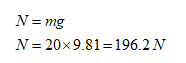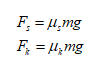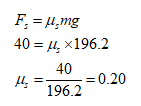# A block of concrete weighs 20 kg, it takes 40 N to start the block sliding on a concrete floor and 10 N to maintain sliding. Calculate the Static coefficiant of friction and calculate the kinetci coefficiant of friction My question is; what is the coefficiant of static and kinetic friction. Ive read throught the book and cant seem to find anything that explains it. I found a formula which reads F = Static coefficiant multiplied by the weight of the block. But that doesnt tell me exactly what the coeffciant of each is, Is it a constant? or does it vary based on the item being moved? Thank you.

Question
1 views

A block of concrete weighs 20 kg, it takes 40 N to start the block sliding on a concrete floor and 10 N to maintain sliding.

Calculate the Static coefficiant of friction and calculate the kinetci coefficiant of friction

My question is; what is the coefficiant of static and kinetic friction. Ive read throught the book and cant seem to find anything that explains it. I found a formula which reads F = Static coefficiant multiplied by the weight of the block. But that doesnt tell me exactly what the coeffciant of each is, Is it a constant? or does it vary based on the item being moved? Thank you.

check_circle

Step 1

Given:

The weight of block of concrete, W = 20 kg

The force required to start the block sliding, Fs = 40 N

The force required to maintain sliding , Fk = 20 N

Step 2

Solution.

The normal force, N is given by the following relation:The coefficient of friction for static and kinetic condition is calculated as :Step 3

For static condition:Thus, the coefficient of static friction is 0.20

...

### Want to see the full answer?

See Solution

#### Want to see this answer and more?

Solutions are written by subject experts who are available 24/7. Questions are typically answered within 1 hour.*

See Solution
*Response times may vary by subject and question.
Tagged in